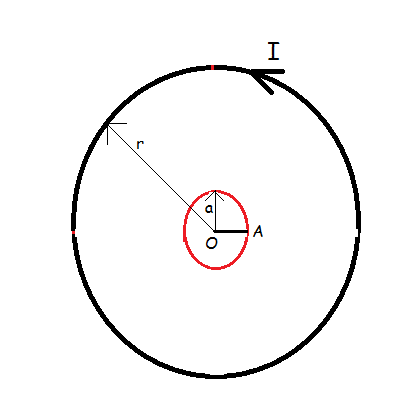# Rotating Rod In Magnetic FieldConsider a horizontal circular loop of radius $r$ carrying a current $I$. Concentric and coplanar with the loop is a smooth metallic ring of radius $a$, containing a metallic rod $OA$ of mass $m$, as shown. The rod can freely rotate about $O$, the center of arrangement. Between $O$ and the circumference of ring, a resistor load $R$(not shown in the figure) is connected, which doesn't obstruct the motion of rod. The rod is given an angular velocity $\omega_{0}$. Find an expression for the maximum angle $\theta$ through which the rod rotates, if $a<.

Find the value of $\displaystyle 2 \Bigg|\bigg(\theta - \frac{16}{3} R m \omega_{0} \bigg(\frac{r}{\mu_{0} I a} \bigg)^2\bigg)\Bigg|$ for the values given below :

Details and assumptions
The resistances of the rod and ring can be neglected.
$m = 1g$
$R = 1 \Omega$
$\omega_{0} = 1 rad/s$
$r = 1m$
$a = 1cm$
$I = 1000 A$
$\mu_{0} = 4 \pi \times 10^{-7} H/m$

×